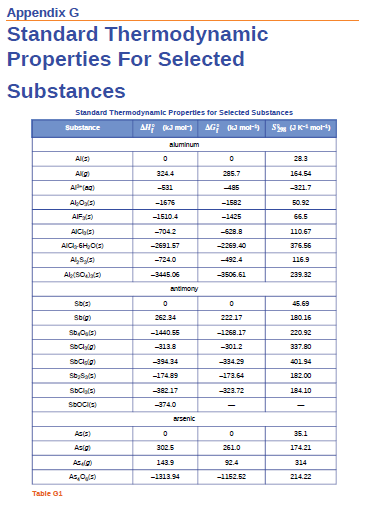# Problem: Use the standard entropy data in Appendix G to determine the change in entropy for each of the reactions listed below. All the processes occur at the standard conditions and 25 °C.(a) MnO2(s) ⟶ Mn(s) + O2(g)(b) H2(g) + Br2(l) ⟶ 2HBr(g)(c) Cu(s) + S(g) ⟶ CuS(s)(d) 2LiOH(s) + CO2(g) ⟶ Li2CO3(s) + H2O(g)(e) CH4(g) + O2(g) ⟶ C(s, graphite) + 2H 2O(g)(f) CS2(g) + 3Cl2(g) ⟶ CCl4(g) + S2Cl2(g)

###### FREE Expert Solution
98% (496 ratings)
###### Problem Details

Use the standard entropy data in Appendix G to determine the change in entropy for each of the reactions listed below. All the processes occur at the standard conditions and 25 °C.

(a) MnO2(s) ⟶ Mn(s) + O2(g)
(b) H2(g) + Br2(l) ⟶ 2HBr(g)
(c) Cu(s) + S(g) ⟶ CuS(s)
(d) 2LiOH(s) + CO2(g) ⟶ Li2CO3(s) + H2O(g)
(e) CH4(g) + O2(g) ⟶ C(s, graphite) + 2H 2O(g)
(f) CS2(g) + 3Cl2(g) ⟶ CCl4(g) + S2Cl2(g)